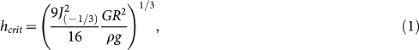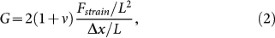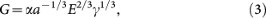## Introduction

The formation of capillary bridges between sand grains are the cause of the stiffness of sculptured wet sand in a sandcastle, as opposed to dry sand which can hardly or not support its own weight1. Qualitatively, the liquid leads to the formation of capillary bridges between the sand grains and the curvature of the liquid interface leads to a capillary pressure causing a force of attraction between the grains. This then creates a network of grains connected by pendular bridges and allows, for example, creating complex structures such as sandcastles. Not many quantitative studies on the mechanical properties of wet sand exist, in spite of the fact that the handling and flow of granular materials is responsible for roughly 10% of the world energy consumption2.

Since in many cases the humidity in the air is sufficient for liquid bridges to form between sand grains, one would expect the mechanical behavior to be well known. This is not the case, in spite of the fact that the stability of wet granular packings is of paramount importance for civil engineering purposes and that the adhesive forces due to the presence of liquid bridges are also extremely important in geophysical applications (i.e., soil stability), of which sandcastles are merely an unusual example3,4,5,6,7,8. For sandcastles, the only estimate in the literature9, argues that the stability is related to the capillary rise in the granular medium and arrives at a maximum sandcastle height of roughly 20 cm. This is in stark disagreement with the observation of sandcastles of several meters high and the common observation that the stability depends on the base radius of the sand structure.

To account for the (in)stability of sandcastles, we show here that it is sufficient to consider that the limit of instability is reached when a column of sand undergoes a buckling transition under its own weight. An elastic rod becomes elastically unstable and buckles under its own weight when exceeding a critical height hcrit10. We present here the analytical solution for hcrit for a cylindrical column:where G is the elastic modulus, R the column radius, ρ is density, g the gravitational acceleration and J ≈ 1.8663 is the smallest positive root of the Bessel function of the first kind of order −1/311. A similar expression is used in civil engineering to calculate the stability of buildings12 and we therefore expect that this also gives the maximum height for which a sandcastle falls apart as the buckling instability will cause the sandcastle to fracture.

## Results

From the buckling arguments, the maximum height varies with the base radius as hmax R2/3. The experimental data for the maximum height as a function of the column radius compare rather favorably with the theoretical expression for buckling (Fig. 2). The exponent of experimental data is in good agreement with theory: hexp R0.7±0.05.

To be able to quantitatively compare with the buckling prediction for the maximum height of a sandcastle, we need to quantify the shear modulus. A recently introduced model for the strength of wet granular matter13 assumes that when one adds a volume of liquid to grains, the capillary attractive force and elastic response from the Hertz contact between two spheres will be balanced. As two beads are always separated by at least the surface roughness, below a critical liquid volume fraction about 0.2%, the bridges between the beads cannot form. At higher volume fractions, the bridge force is dominated by the curvature of the meniscus and at even higher volume fractions the bridges start merge into larger pockets of fluid13. The macroscopic shear modulus G of a macroscopic cube of dimension L containing a large amount of grains can be defined as the ratio of stress and strain:where Δx/L is the strain, Fstrain/L2 the stress and ν ≈ 0.5 the Poisson ratio. Assuming that at the level of single particles the capillary and elastic forces are balanced for each pair of grains and using the simple Hertz contact for the grain contact elasticity, the optimum strength G can be found by averaging over all pairs as13:where a is the radius of the grains, E is the Young's modulus of the grain material and γ is the surface tension of the liquid-air interface, respectively. α is constant of proportionality that expresses how much the individual capillary sphere-sphere bonds are deformed relative to the globally imposed strain. To compute an estimate of α, we take a simple cubic crystal of frictionless spheres and average α over different straining directions, which gives α ≈ 0.054. We take the function f(Vf) ≈ 1 for determining the maximum strength; f(Vf) shows the dependence of the elastic modulus to the liquid volume fraction and is unity for the optimum volume fraction13.

## Discussion

This model gives a very accurate result for the maximum strength of the sand packing (Fig. 3), which makes it possible to compute how high sandcastles can be built from the predicted elastic modulus for any size grains of wet sand. Using typical values for beach sand, a cylinder with a radius of 20 cm for instance could be as tall as about 2.5 m, which is in quite good agreement with what can be observed for real sandcastles14. This estimate is an immense improvement compared to a previous dimensional analysis result which gives roughly 20 cm as the maximum height of a sandcastle, independently of the base diameter9. For our cylindrical sandcastles, using the optimum strength in the buckling arguments, we arrive at a quantitative theory for sandcastle stability that agrees with the measurements (Fig. 2).